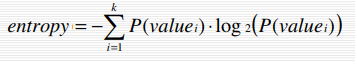Search

# What is Entropy and Information Gain? How are they used to construct decision trees?

Decision tree is one of the simplest and common Machine Learning algorithms, that are mostly used for predicting categorical data. Entropy and Information Gain are 2 key metrics used in determining the relevance of decision making when constructing a decision tree model.

Let’s try to understand what the “Decision tree” algorithm is.

So, what is a Decision tree?

If we strip down to the basics, decision tree algorithms are nothing but a series of if-else statements that can be used to predict a result based on a dataset. This flowchart-like structure helps us in decision making.

The idea of a decision tree is to divide the data set into smaller data sets based on the descriptive features until we reach a small enough set that contains data points that fall under one label.

Each feature of the data set becomes a root[parent] node, and the leaf[child] nodes represent the outcomes. For instance, this is a simple decision tree that can be used to predict whether I should write this blog or not.Image by Author

Such a simple decision making is also possible with decision trees. They are easy to understand and interpret because they mimic human thinking.

Alright, now let’s see what is Entropy and Information Gain and how they are used to construct decision trees.

## What is Entropy?

Entropy is the measures of impurity, disorder or uncertainty in a bunch of examples. Entropy controls how a Decision Tree decides to split the data. The below image shows impurity level of each set.Image by Author

If we have a set of K different values , then we can calculate the entropy using this formula:where, P(valuei ) is the probability of getting the ith value when randomly selecting one from the set.

For eg, let’s take the following image with green and red circles.Image by Author

In this group, we have 14 circles, out of which 10 are green (10/14) and 4 are red (4/14). Let’s find the entropy of this group.Note:

• The entropy of a group in which all examples belong to the same class will always be 0 as shown below:Image by Author
• The entropy of a group with 50% in either class will always be 1 as shown below:Image by Author

## What is Information Gain?

Information gain (IG) measures how much “information” a feature gives us about the class.It tells us how important a given attribute of the feature vectors is. Information gain (IG) is used to decide the ordering of attributes in the nodes of a decision tree.

Information gain (IG) is calculated as follows:

Information Gain = entropy(parent) – [average entropy(children)]

Let’s look at an example to demonstrate how to calculate Information Gain.

Let's say a set of 30 people both Male and female are split according to their age. Each person’s age is compared to 30 and they are separated into 2 child groups as shown in the image and their corresponding node’s entropy is calculated. The main node is called the Parent node and the 2 sub nodes are called child nodes.Image by Author

The entropies of parent and child nodes are calculated as shown below. The Information gain is then calculated using the entropy of individual nodes.The steps that needs to be followed to construct a decision tree using Information gain is shown below:Image by Author

Entropy and Information Gain are two main concepts that are used when it comes to constructing a decision tree, to determine the nodes and the best way to split.

You may also want to review my blog on Gini Impurity, another important concept/method used to construct decision trees.

Hope this will be helpful for everyone who wants to work on decision trees.

Happy decision making!

2,021 views1 comment

See All